# Density-functional theory

Density-functional theory is a set of theories in statistical mechanics that profit from the fact that the Helmholtz energy function of a system can be cast as a functional of the density. That is, the density (in its usual sense of particles per volume), which is a function of the position in inhomogeneous systems, uniquely defines the Helmholtz energy. By minimizing this Helmholtz energy one arrives at the true Helmholtz energy of the system and the equilibrium density function. The situation parallels the better known electronic density functional theory, in which the energy of a quantum system is shown to be a functional of the electronic density (see the theorems by Hohenberg, Kohn, Sham, and Mermin).

Starting from this fact, approximations are usually made in order to approach the true functional of a given system. An important division is made between local and weighed theories. In a local density theory the in which the dependence is local, as exemplified by the (exact) Helmholtz energy of an ideal system: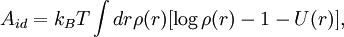$A_{id}=k_BT\int dr \rho(r) [\log \rho(r) -1 -U(r)],$

where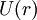$U(r)$ is an external potential. It is an easy exercise to show that Boltzmann's barometric law follows from minimization. An example of a weighed density theory would be the (also exact) excess Helmholtz energy for a system of 1-dimensional hard rods: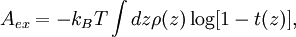$A_{ex}=-k_BT\int dz \rho(z) \log [1-t(z)],$

where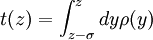$t(z)=\int_{z-\sigma}^z dy \rho(y)$, precisely an average of the density over the length of the hard rods,$\sigma$. "Excess" means "over ideal", i.e., it is the total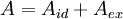$A=A_{id}+A_{ex}$ that is to be minimized.